Jan 15, 2023

# Building an FPGA Computer: SAP-2

Previously I built the SAP-1 on an FPGA based on a design laid out in the book Digital Computer Electronics by Malvino and Brown. Now it’s time to build the SAP-2, also from the book, which is quite a large step up from the SAP-1.

While the SAP-1 was explained in great detail in the book, the SAP-2 is much more vague and undefined. They don’t go into implementation details, provide schematics, or show the control signals. Instead they give a brief functional overview of its components, list out its instructions, and that’s about it. Thus I was forced to figure things out on my own, for better or for worse.

When creating my FPGA version, I decided to be content with it being functionally identical without getting hung up on the implementation details. As such, a program run on their theoretical version would have the same output as on mine, but the way it got there and the time it took to do it would be different.

This post is intended as a supplement to the first so I’ll assume readers of this one are familiar already with the SAP-1 to save me from repeating myself. I’ll refer back to it and mention ways that the SAP-2 differs.

If you find the architecture of this version to be highly awkward and strange, we are in agreement.

The SAP-2 is akin to a teenager lying somewhere between the toddler SAP-1 and the adult SAP-3. While the SAP-1 and SAP-3 can stand on their own, the SAP-2 is trying to bridge the gap between them and does so quite poorly.

I didn't want to do the SAP-1 and then skip to the SAP-3, so my only choice was to recreate the behavior as written in the book, which made for some very awkward Verilog code.

## Overview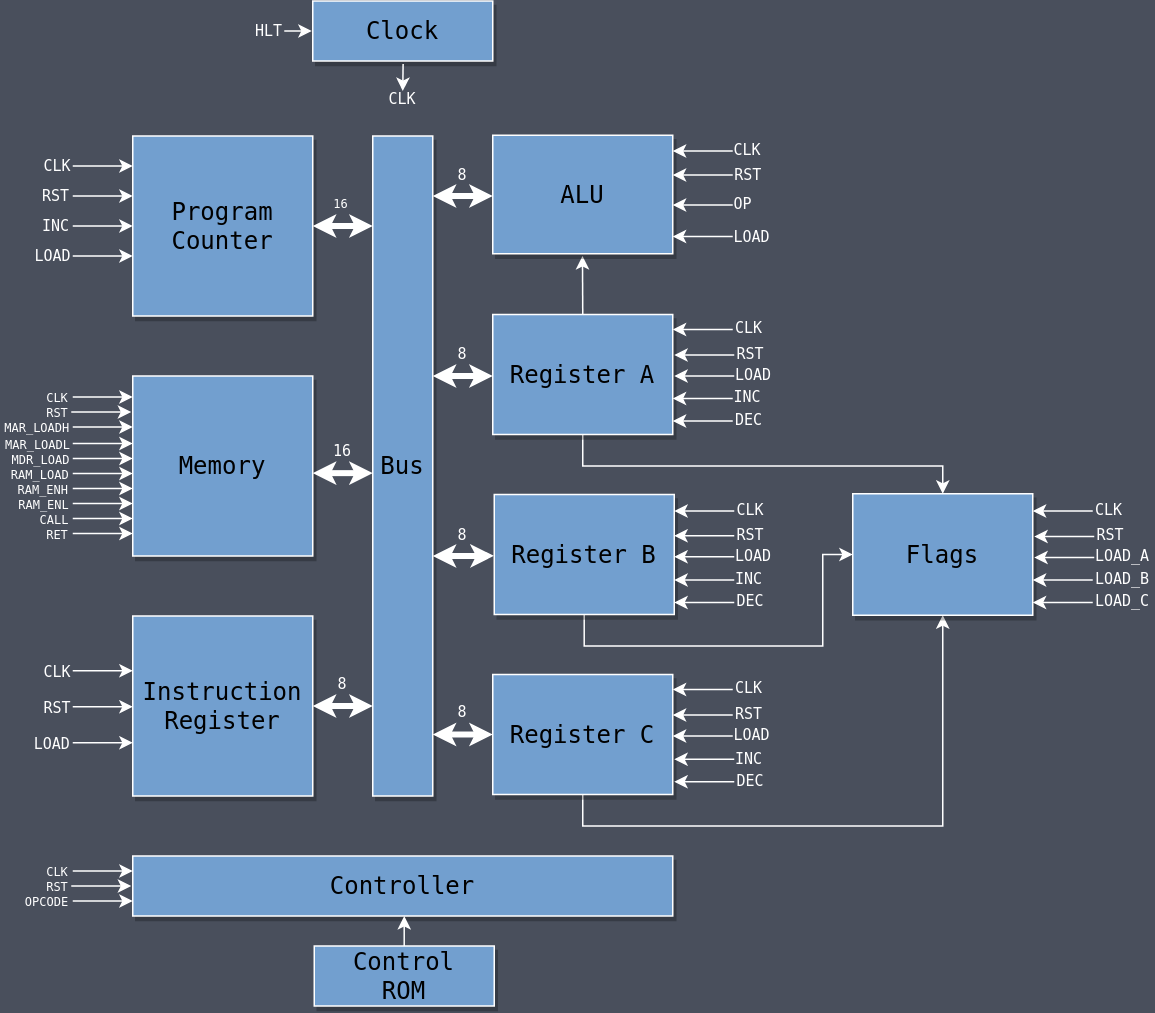## Modules

### Clock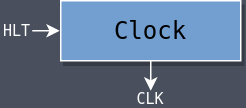The clock is unchanged from the SAP-1.

 ``````1 2 3 4 5 6 7 8 9 `````` ``````module clock( input hlt, input clk_in, output clk_out ); assign clk_out = (hlt) ? 1'b0 : clk_in; endmodule ``````

### Program Counter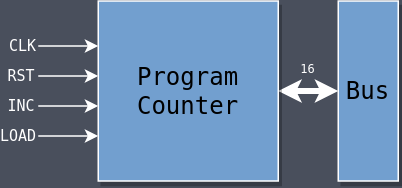The program counter has been widened to 16 bits and it has an additional load signal.

The load signal loads the PC with a value taken from the bus, a requirement for being able to jump around during a program’s execution.

 `````` 1 2 3 4 5 6 7 8 9 10 11 12 13 14 15 16 17 18 19 20 21 22 23 24 `````` ``````module pc( input clk, input rst, input inc, input load, input[15:0] bus, output[15:0] out ); reg[15:0] pc; always @(posedge clk, posedge rst) begin if (rst) begin pc <= 16'b0; end else if (load) begin pc <= bus; end else if (inc) begin pc <= pc + 1; end end assign out = pc; endmodule ``````

### Registers A/B/C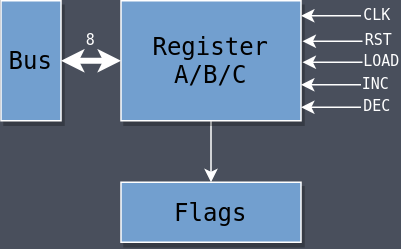There are now three registers: A, B, and C. Each are functionally identical so there is a single register module that is used for all three. It’s instantiated three times in the top module and given different names for each.

The book briefly mentions that register increments and decrements are done using the ALU, which would require putting a 1 into the ALU’s temp register, putting the contents of B or C into A, and then doing an addition or a subtraction. But it doesn’t explain where that 1 comes from, how to avoid the A register being overwritten for increments and decrements of B or C, or how it’s supposed to be done in only four T-states.

I instead opted to give each register an inc and a dec signal to increment and decrement, respectively, rather than go through the ALU. I may figure out a way to use the ALU directly when it comes time to build the SAP-3.

 `````` 1 2 3 4 5 6 7 8 9 10 11 12 13 14 15 16 17 18 19 20 21 22 23 24 25 26 27 `````` ``````module register( input clk, input rst, input load, input inc, input dec, input[15:0] bus, output[7:0] out ); reg[7:0] data; always @(posedge clk, posedge rst) begin if (rst) begin data <= 8'b0; end else if (load) begin data <= bus[7:0]; end else if (inc) begin data <= data + 1; end else if (dec) begin data <= data - 1; end end assign out = data; endmodule ``````

### ALU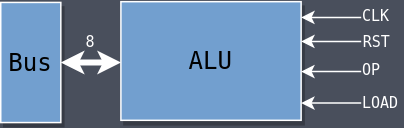The Arithmetic Logic Unit (ALU) is the same as the SAP-1’s Adder but with the ability to do logic operations.

The temporary register has been moved inside of the ALU module itself which can be loaded with the load signal which frees up Register B for other uses. Register A continues to be the primary register for operations, using the temp register as the other operand when required. The control logic is responsible for filling the temp register with the necessary data prior to doing the operation.

The ALU can perform eight operations:

• Subtraction (SUB)
• Logical AND (AND)
• Logical OR (OR)
• Logical XOR (XOR)
• Complement (CMA)
• Rotate All Left (RAL)
• Rotate All Right (RAR)

An arithmetic shift left will move the bits that are shifted out the left side into the right side, while an arithmetic shift right will move zeroes into the left side.

The operation to be performed is determined by the op signal.

 `````` 1 2 3 4 5 6 7 8 9 10 11 12 13 14 15 16 17 18 19 20 21 22 23 24 25 26 27 28 29 30 31 32 33 34 35 36 37 38 39 40 41 42 43 44 45 46 47 48 49 50 51 52 53 54 55 56 57 58 59 60 61 62 63 64 65 66 `````` ``````module alu( input clk, input rst, input[7:0] a, input[2:0] op, input load, input[15:0] bus, output[7:0] out ); reg[7:0] alu; reg[7:0] tmp; localparam OP_ADD = 0; localparam OP_SUB = 1; localparam OP_AND = 2; localparam OP_OR = 3; localparam OP_XOR = 4; localparam OP_CMA = 5; localparam OP_RAL = 6; localparam OP_RAR = 7; always @(posedge clk, posedge rst) begin if (rst) begin tmp <= 8'b0; end else if (load) begin tmp <= bus[7:0]; end end always @(*) begin case (op) OP_ADD: begin alu = a + tmp; end OP_SUB: begin alu = a - tmp; end OP_AND: begin alu = a & tmp; end OP_OR: begin alu = a | tmp; end OP_XOR: begin alu = a ^ tmp; end OP_CMA: begin alu = ~a; end OP_RAL: begin alu = a << 1; alu = a; end OP_RAR: begin alu = a >> 1; end default: begin alu = 0; end endcase end assign out = alu; endmodule ``````

### Flags Register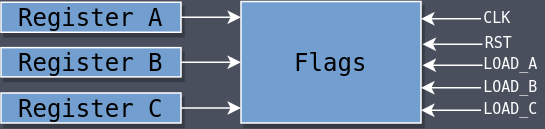The flags register is a special register used to hold the status of previous operations. There are two:

• Zero Flag: Set if the previous operation caused a result of 0.
• Sign Flag: Set if the previous operation caused a negative result.

It’s a bit unusual that the flags register is connected to A/B/C directly instead of to the ALU, but that was required so that the INR and DCR instructions would update the flags without going through the ALU, as discussed above.

Not all instructions should update the flags so there are three signals that dictate whether or not the flags are updated based on the contents of each of the three registers: load_a, load_b, and load_c.

For example, INR C operates on Register C, so the control logic asserts load_c so that the flags are updated based on the contents of C. If the increment operation causes C to become zero then FLAG_Z is set. If the operation causes C to become negative then FLAG_S is set.

But the instruction MVI C, A, which copies the contents of A into C, should not update the flags, and so the load_c signal is not asserted during that instruction.

 `````` 1 2 3 4 5 6 7 8 9 10 11 12 13 14 15 16 17 18 19 20 21 22 23 24 25 26 27 28 29 30 31 32 33 34 35 `````` ``````module flags( input clk, input rst, input[7:0] a, input[7:0] b, input[7:0] c, input load_a, input load_b, input load_c, output[1:0] out ); localparam FLAG_Z = 1; localparam FLAG_S = 0; reg[1:0] flags; always @(negedge clk, posedge rst) begin if (rst) begin flags <= 2'b0; end else if (load_a) begin flags[FLAG_Z] <= (a == 0) ? 1'b1 : 1'b0; flags[FLAG_S] <= (a == 1) ? 1'b1 : 1'b0; end else if (load_b) begin flags[FLAG_Z] <= (b == 0) ? 1'b1 : 1'b0; flags[FLAG_S] <= (b == 1) ? 1'b1 : 1'b0; end else if (load_c) begin flags[FLAG_Z] <= (c == 0) ? 1'b1 : 1'b0; flags[FLAG_S] <= (c == 1) ? 1'b1 : 1'b0; end end assign out = flags; endmodule ``````

### Memory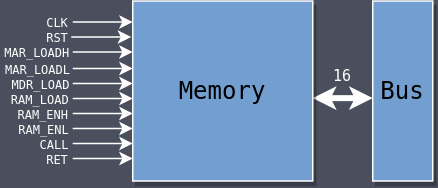The memory has been expanded to 64K which requires a 16-bit address space to be able to address from 0x0000 to 0xFFFF. The expansion to 16-bit requires more complicated control logic which requires more control signals.

There is also a new internal register called the Memory Data Register (MDR) which holds values temporarily when reading from or writing to the memory. mdr_load loads into the MDR from the bus and ram_load loads into memory from the MDR.

The MDR is 16-bit even though data values should only be 8-bit because certain instructions (namely LDA and STA) require holding two separate values in the MDR at one time. The high byte is loaded with ram_enh and the low byte is loaded with ram_enl. I’m not sure if this was what Malvino and Brown had in mind but it works.

The call signal loads two special memory locations (0xFFFE and 0xFFFF) with the contents of the bus and the ret signal loads from those two locations into the MDR to be later put back into the PC. These two signals are used to facilitate the CALL and RET instructions which will be discussed later. The implication of this rudimentary system is that a function cannot call another function or else the return address will be overwritten. The SAP-3 will fix that.

 `````` 1 2 3 4 5 6 7 8 9 10 11 12 13 14 15 16 17 18 19 20 21 22 23 24 25 26 27 28 29 30 31 32 33 34 35 36 37 38 39 40 41 42 43 44 45 46 47 48 49 50 51 52 53 54 55 56 57 58 59 `````` ``````module memory( input clk, input rst, input mar_loadh, input mar_loadl, input mdr_load, input ram_load, input ram_enh, input ram_enl, input call, input ret, input[15:0] bus, output[15:0] out ); initial begin \$readmemh("program.bin", ram); end reg[15:0] mar; reg[15:0] mdr; reg[7:0] ram[0:65535]; always @(posedge rst) begin mar <= 16'b0; mdr <= 16'b0; end always @(posedge clk) begin if (mar_loadh) begin mar[15:8] <= bus[15:8]; end if (mar_loadl) begin mar[7:0] <= bus[7:0]; end if (mdr_load) begin mdr[7:0] <= bus[7:0]; end if (ret) begin mdr[15:8] <= ram[16'hFFFE]; mdr[7:0] <= ram[16'hFFFF]; end else if (call) begin ram[16'hFFFE] <= bus[15:8]; ram[16'hFFFF] <= bus[7:0]; end else if (ram_enh) begin mdr[15:8] <= ram[mar]; end else if (ram_enl) begin mdr[7:0] <= ram[mar]; end else if (ram_load) begin ram[mar] <= mdr; end end assign out = mdr; endmodule ``````

### Instruction Register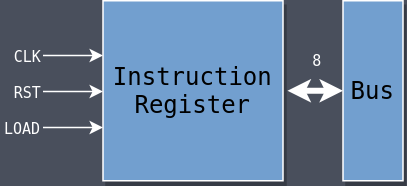The Instruction Register (IR) is unchanged from the SAP-1 except that it only uses the lower eight bits of the bus during a load.

 `````` 1 2 3 4 5 6 7 8 9 10 11 12 13 14 15 16 17 18 19 20 21 `````` ``````module ir( input clk, input rst, input load, input[15:0] bus, output[7:0] out ); reg[7:0] ir; always @(posedge clk, posedge rst) begin if (rst) begin ir <= 8'b0; end else if (load) begin ir <= bus[7:0]; end end assign out = ir; endmodule ``````

### Instructions

There are 39 instructions which is a lot more than the SAP-1. The book also includes an IN and an OUT but I removed those because they don’t make sense for the version I’m building.

The book uses the same opcodes for the instructions as the Intel 8080 and I did the same to stay consistent. The authors provide the number of T-states that are required for each instruction but I was unable to reach the same values for many of the instructions. In some cases my implementation uses fewer T-states and in some others it uses more.

In particular, the INR and DCR instructions don’t make sense as described by Malvino and Brown. They claim that those two instructions use Register A for those operations but if that were true then the contents of A would be lost and it would take a lot more than four T-states as they claim. I decided to use explicit inc and dec signals for each of the three main registers to allow for directly incrementing and decrementing them in a single T-state.

Some instructions update the flags and some do not. If an instruction operates on a particular register then the flags are updated based on the conditions of that register (e.g., INR B will update the flags if B becomes 0 or negative).

• Direct
• Required data is located at memory address which follows the opcode
• Immediate
• Required data is specified in the instruction itself
• Register
• Required data is inside of a register
• Implied
• Required data is implied by the opcode

Every instruction takes at least three T-states because it takes three just to fetch the instruction from memory. The instructions with the most T-states are those that deal deal with memory addresses because of the time required to shuffle addresses from the PC to the MAR and MDR.

JM, JNZ, and JZ take a variable number of cycles depending on whether the jump occurs or not. If the jump occurs it takes eight T-states, otherwise it takes four.

The length of my instructions differ from those in the book and are faster in many places, but not all. I tried to think of solutions that would match theirs exactly but was unable to figure out how they get their numbers.

ADD B805S,ZRegister1A = A + B
ADD C815S,ZRegister1A = A + C
ANA BA05S,ZRegister1A = A & B
ANA CA15S,ZRegister1A = A & C
ANI byteE67S,ZImmediate2A = A & byte
CMA2F4-Implied1A = ~A
DCR A3D4S,ZRegister1A = A - 1
DCR B054S,ZRegister1B = B - 1
DCR C0D4S,ZRegister1C = C - 1
HLT764--1Halt execution
INR A3C4S,ZRegister1A = A + 1
INR B044S,ZRegister1B = B + 1
INR C0C4S,ZRegister1C = C + 1
MOV A, B784-Register1A = B
MOV A, C794-Register1A = C
MOV B, A474-Register1B = A
MOV B, C414-Register1B = C
MOV C, A4F4-Register1C = A
MOV C, B484-Register1C = B
MVI A, byte3E6-Immediate2A = byte
MVI B, byte066-Immediate2B = byte
MVI C, byte0E6-Immediate2C = byte
NOP003--1Do nothing
ORA BB05S,ZRegister1A = A
ORA CB15S,ZRegister1A = A
ORI byteF67S,ZImmediate2A = A
RAL174-Implied1A « 1, LSB becomes zero
RAR1F4-Implied1A » 1, MSB goes to LSB
RETC95-Implied1Return from function
SUB B905S,ZRegister1A = A - B
SUB C915S,ZRegister1A = A - C
XRA BA85S,ZRegister1A = A ^ B
XRA CA95S,ZRegister1A = A ^ C
XRI byteEE7S,ZImmediate2A = A ^ byte

### Bus

The bus has been widened to 16 bits to accommodate the memory address space and, like the SAP-1, a multiplexor is used to select which module gets to output onto the bus at any one time.

 `````` 1 2 3 4 5 6 7 8 9 10 11 12 13 14 15 16 17 18 19 `````` ``````reg[15:0] bus; always @(*) begin if (a_en) begin bus = a_out; end else if (b_en) begin bus = b_out; end else if (c_en) begin bus = c_out; end else if (alu_en) begin bus = alu_out; end else if (pc_en) begin bus = pc_out; end else if (mdr_en) begin bus = mem_out; end else begin bus = 16'b0; end end ``````

### Controller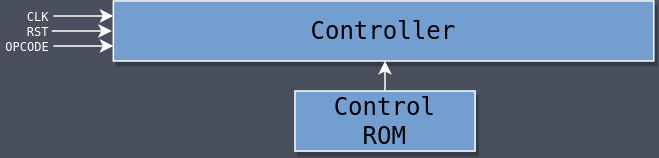The SAP-2 has 35 control signals (23 more than the SAP-1) and the longest instruction takes 10 T-states (four more than the longest SAP-1 instruction). Trying to implement all of that logic with a switch statement like we did for the SAP-1 would result in a massive module that would be hard to write, hard to understand, and hard to debug.

Fortunately control logic is combinational logic, and all combinational logic can be represented in the form of Read-Only Memory (ROM) by using the inputs to the combinational logic to address into the ROM. The value stored at that address is the output corresponding to those inputs.

Every instruction is one byte (eight bits) and the maximum required number of T-states is 10 (requiring four bits to represent). We can combine those together into a single 12-bit address. The instruction goes into bits 11-4 and the T-state value goes into bits 3-0: IIIIIIII TTTT. Then, if we want to know what control signal should be asserted for a given instruction and certain T-state, we can look it up in the ROM.

For example, if the instruction is ADD B (\$80) and we’re on stage 3, we concatenate \$80 and \$3 together to get address \$803. The value at that address is the 35-bit control word detailing which signals to assert at that point in time. In this case: 00000001000000000000100000000000000, where the two asserted signals are B_EN and ALU_LOAD.

The question is: how to fill out the ROM? A 12-bit address means 4096 different values to program which would be a major pain (and error-prone) to do by hand. Instead I used a spreadsheet where the rows are the addresses and the columns are the different control signals and used the CONCATENATE() function to concatenate all of the signal bits into a single string. Then it was just a matter of copying that entire binary string column into a file which could be loaded in Verilog using readmemb.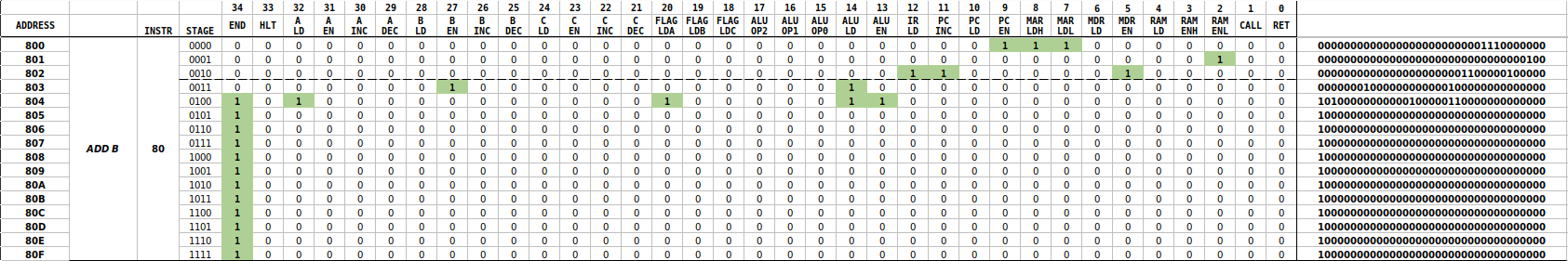A lot of space is wasted because because the 12-bit address allows for 256 instructions but there are only 41. If the SAP-2 wasn’t using a subset of the Intel 8080 instruction set then the instruction op codes could start at \$00 and go up to \$25, reducing the number of address lines required to six. But using the 8080 instruction set means we can use existing 8080 programs which is useful.

Given that the control ROM contains all of the logic, the actual controller itself doesn’t do much anymore. Mostly it just handles the special logic required for the jump instructions, where it checks the flag states if the current instruction is JZ, JNZ, or JM, and if it’s currently in stage 4, which is where a decision needs to be made.

Other than that, it increments the stage like usual, and pulls the current control word out of the ROM based on the current instruction and stage acting as the address.

There is also a special signal called END which is used to reset the stage back to 0 when an instruction has finished so that all 16 possible T-states don’t have to cycle through before the next instruction begins. Every instruction ends with that signal asserted, as shown in the spreadsheet image above.

 `````` 1 2 3 4 5 6 7 8 9 10 11 12 13 14 15 16 17 18 19 20 21 22 23 24 25 26 27 28 29 30 31 32 33 34 35 36 37 38 39 40 41 42 43 44 45 46 47 48 49 50 51 52 53 54 55 56 57 58 59 60 61 62 63 64 65 66 67 68 69 70 71 72 73 74 75 76 77 78 79 80 81 82 83 84 85 86 87 88 `````` ``````module controller( input clk, input rst, input[7:0] opcode, input[1:0] flags, output[33:0] out ); localparam SIG_END = 34; localparam SIG_HLT = 33; localparam SIG_A_LOAD = 32; localparam SIG_A_EN = 31; localparam SIG_A_INC = 30; localparam SIG_A_DEC = 29; localparam SIG_B_LOAD = 28; localparam SIG_B_EN = 27; localparam SIG_B_INC = 26; localparam SIG_B_DEC = 25; localparam SIG_C_LOAD = 24; localparam SIG_C_EN = 23; localparam SIG_C_INC = 22; localparam SIG_C_DEC = 21; localparam SIG_FLAGS_LDA = 20; localparam SIG_FLAGS_LDB = 19; localparam SIG_FLAGS_LDC = 18; localparam SIG_ALU_OP2 = 17; localparam SIG_ALU_OP1 = 16; localparam SIG_ALU_OP0 = 15; localparam SIG_ALU_LD = 14; localparam SIG_ALU_EN = 13; localparam SIG_IR_LOAD = 12; localparam SIG_PC_INC = 11; localparam SIG_PC_LOAD = 10; localparam SIG_PC_EN = 9; localparam SIG_MAR_LOADH = 8; localparam SIG_MAR_LOADL = 7; localparam SIG_MDR_LOAD = 6; localparam SIG_MDR_EN = 5; localparam SIG_RAM_LOAD = 4; localparam SIG_RAM_ENH = 3; localparam SIG_RAM_ENL = 2; localparam SIG_CALL = 1; localparam SIG_RET = 0; localparam OP_JZ = 8'hCA; localparam OP_JNZ = 8'hC2; localparam OP_JM = 8'hFA; localparam FLAG_Z = 1; localparam FLAG_S = 0; reg[34:0] ctrl_word; reg[34:0] ctrl_rom[0:4095]; initial begin \$readmemb("ctrl_rom.bin", ctrl_rom); end reg[3:0] stage; always @(negedge clk, posedge rst) begin if (rst) begin stage <= 0; end else begin if (stage_rst) begin stage <= 0; end else begin stage <= stage + 1; end end end reg stage_rst; always @(*) begin ctrl_word = ctrl_rom[{opcode, stage}]; if ((opcode == OP_JZ && stage == 4 && flags[FLAG_Z] == 0) || (opcode == OP_JNZ && stage == 4 && flags[FLAG_Z] == 1) || (opcode == OP_JM && stage == 4 && flags[FLAG_S] == 0)) begin stage_rst = 1; end else begin stage_rst = ctrl_rom[{opcode, stage}][SIG_END]; end end assign out = ctrl_word; endmodule ``````

## Programming

The SAP-2 uses a subset of the Intel 8080 instruction set which has the nice benefit of being able to use existing 8080 tools for our version, like an assembler. The Pretty 8080 Assembler lets you write 8080 assembly and then assembles it into various forms for you.

Here’s a perfectly valid 8080 program written in assembly using only the subset of instructions listed above:

 `````` 1 2 3 4 5 6 7 8 9 10 11 12 `````` ``````MVI A, 0 ; A = 0 MVI B, 12 ; B = 12 MVI C, 8 ; C = 8 REPEAT: ADD B ; A += B DCR C ; C-- JZ DONE ; if (C == 0) go to DONE JMP REPEAT ; else go to REPEAT DONE: HLT ``````

Here it is annotated with addresses, opcodes, and operands:

 `````` 1 2 3 4 5 6 7 8 9 10 11 12 `````` ``````\$0000 | 3E 00 | MVI A, 0 ; A = 0 \$0002 | 06 0C | MVI B, 12 ; B = 12 \$0004 | 0E 08 | MVI C, 8 ; C = 8 | | | | REPEAT: \$0006 | 80 | ADD B ; A += B \$0007 | 0D | DCR C ; C-- \$0008 | CA 0E 00 | JZ DONE ; if (C == 0) go to DONE \$000B | C3 06 00 | JMP REPEAT ; else go to REPEAT | | | | DONE: \$000E | 76 | HLT ``````

Using the HEX button in the assembler will write out the program as hex values to a file on disk, but unfortunately it also includes extra characters we don’t want. Instead we can use the BIN button to write out actual binary data instead. Then we can use a tool called hexdump to convert that to hex for us which we can then read into memory inside of Verilog.

 ``````1 `````` ``````hexdump -ve '8/1 "%02x " "\n"' program.com > program.bin ``````

## Simulation

Again we use a testbench to simulate the design and verify correctness.

 `````` 1 2 3 4 5 6 7 8 9 10 11 12 13 14 15 16 17 18 19 20 21 22 23 24 25 26 27 28 29 30 31 32 33 34 35 36 37 38 39 40 41 42 43 44 45 46 47 48 49 50 51 52 53 54 55 56 57 58 59 60 61 62 63 64 65 66 67 68 69 70 71 72 73 74 75 76 77 78 79 80 81 82 83 84 85 86 87 88 89 90 91 92 93 94 95 96 97 98 99 100 101 102 103 104 105 106 107 108 109 110 111 112 113 114 115 116 117 118 119 120 121 122 123 124 125 126 127 128 129 130 131 132 133 134 135 136 137 138 139 140 141 142 143 144 145 146 147 148 149 150 151 152 153 154 155 156 157 158 159 160 161 162 163 164 165 166 167 168 169 170 171 172 173 174 175 176 177 178 179 180 181 182 183 184 185 186 187 188 189 190 191 `````` ``````module top_tb(); initial begin \$dumpfile("top_tb.vcd"); \$dumpvars(0, top_tb); rst = 1; #1 rst = 0; end reg[15:0] bus; always @(*) begin if (a_en) begin bus = a_out; end else if (b_en) begin bus = b_out; end else if (c_en) begin bus = c_out; end else if (alu_en) begin bus = alu_out; end else if (pc_en) begin bus = pc_out; end else if (mdr_en) begin bus = mem_out; end else begin bus = 16'b0; end end reg clk_in = 0; integer i; initial begin for (i = 0; i < 512; i++) begin #1 clk_in = ~clk_in; end end reg rst; wire hlt; wire clk; clock clock( .hlt(hlt), .clk_in(clk_in), .clk_out(clk) ); wire pc_inc; wire pc_load; wire pc_en; wire[15:0] pc_out; pc pc( .clk(clk), .rst(rst), .inc(pc_inc), .load(pc_load), .bus(bus), .out(pc_out) ); wire ir_load; wire[7:0] ir_out; ir ir( .clk(clk), .rst(rst), .load(ir_load), .bus(bus), .out(ir_out) ); wire mar_loadh; wire mar_loadl; wire mdr_load; wire mdr_en; wire ram_load; wire ram_enh; wire ram_enl; wire call; wire ret; wire[15:0] mem_out; memory mem( .clk(clk), .rst(rst), .mar_loadh(mar_loadh), .mar_loadl(mar_loadl), .mdr_load(mdr_load), .ram_load(ram_load), .ram_enh(ram_enh), .ram_enl(ram_enl), .call(call), .ret(ret), .bus(bus), .out(mem_out) ); wire a_load; wire a_en; wire a_inc; wire a_dec; wire[7:0] a_out; register reg_a( .clk(clk), .rst(rst), .load(a_load), .inc(a_inc), .dec(a_dec), .bus(bus), .out(a_out) ); wire b_load; wire b_en; wire b_inc; wire b_dec; wire[7:0] b_out; register reg_b( .clk(clk), .rst(rst), .load(b_load), .inc(b_inc), .dec(b_dec), .bus(bus), .out(b_out) ); wire c_load; wire c_en; wire c_inc; wire c_dec; wire[7:0] c_out; register reg_c( .clk(clk), .rst(rst), .load(c_load), .inc(c_inc), .dec(c_dec), .bus(bus), .out(c_out) ); wire[2:0] alu_op; wire alu_load; wire alu_en; wire[7:0] alu_out; alu alu( .clk(clk), .rst(rst), .a(a_out), .load(alu_load), .op(alu_op), .bus(bus), .out(alu_out) ); wire[1:0] flags_out; wire flags_lda; wire flags_ldb; wire flags_ldc; flags flags( .clk(clk), .rst(rst), .a(a_out), .b(b_out), .c(c_out), .load_a(flags_lda), .load_b(flags_ldb), .load_c(flags_ldc), .out(flags_out) ); controller controller( .clk(clk), .rst(rst), .opcode(ir_out), .flags(flags_out), .out({ hlt, a_load, a_en, a_inc, a_dec, b_load, b_en, b_inc, b_dec, c_load, c_en, c_inc, c_dec, flags_lda, flags_ldb, flags_ldc, alu_op, alu_load, alu_en, ir_load, pc_inc, pc_load, pc_en, mar_loadh, mar_loadl, mdr_load, mdr_en, ram_load, ram_enh, ram_enl, call, ret}) ); endmodule ``````

Simulation generates the following waveforms, where the final value of A is 96 as expected: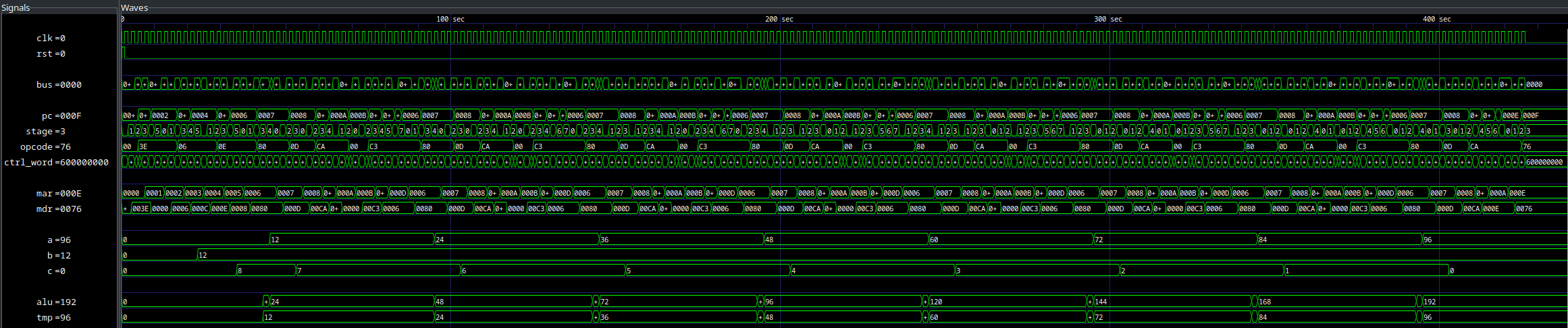## Source Code

You can find all of the code here.

Last Edited: Feb 27, 2023